• 根据dataframe某列或多列进行拆分，获得小dataframe后从大到小排序，取前90%，再合并成一个大的dataframe

日拱一卒，功不唐捐

知识点

exec()
enumerate()
isin()
concat()

新创建DataFrame
import datetime
import pandas as pd
import numpy as np

#定义list拓展函数
def list_extend(_list,times):
OrginList=[_list for _i in range(times)]

_extendList=[]

for k,j in enumerate(OrginList):
for i in j:
_extendList.append(i)

return _extendList

# 定义日期循环函数
def Date(start,end):
_date=[]
for i in range((end-start).days+1):
_date.append(start + datetime.timedelta(days=i))
return _date

#创建DataFrame
ShopName=["Sam's Club",'bravo','WU MART','Vangguard']
start=datetime.date(2019,1,1)
end=datetime.date(2019,6,1)

data = pd.DataFrame({'ShopName':list_extend(ShopName,38),
'income':random.sample(list(range(0,200)),152),
'date':Date(start,end)})
data.head(3)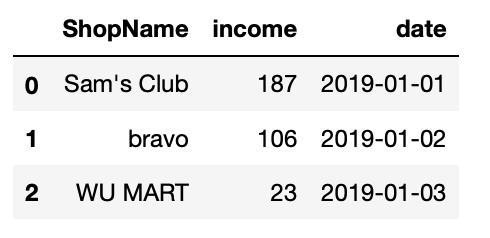1. 根据某列值拆分成多个表
对商品进行分组，获取小dataframe
for shop_index,temp_shop_info in enumerate(shop_info):
#对ShopName商铺进行拆分
temp_shop_data=data[data['ShopName'].isin([temp_shop_info])]
exec("df%s=temp_shop_data"%shop_index)

df1.head(4)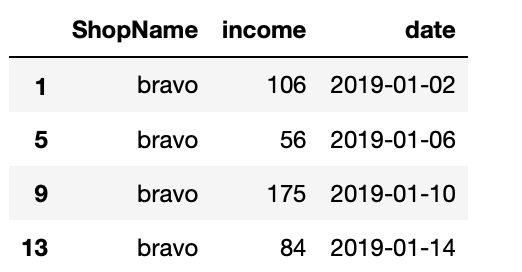2. 根据多列值拆分成多个表
先对商品进行分组，再对月份进行分组，获取小dataframe
for shop_index,temp_shop_info in enumerate(shop_info):
#对ShopName商铺进行拆分
temp_shop_data=data[data['ShopName'].isin([temp_shop_info])]
for month_index,temp_month_info in enumerate(month_info):
#对month进行拆分
temp_month_date=temp_shop_data[temp_shop_data['month'].isin([temp_month_info])]
exec("df%s=temp_month_date"%month_index)

df0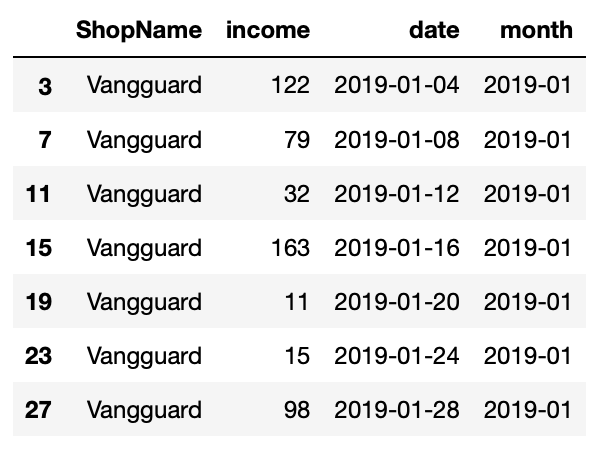3. 多个DataFrame合并成一个大的DataFrame
先对商品进行分组，再对月份进行分组，获取小dataframe，对income值从大到小排序，取前90%数据后获得新的dataframe，再把所有小dataframe合并成一个大的dataframe
df_frames=[]
for shop_index,temp_shop_info in enumerate(shop_info):
#对ShopName商铺进行拆分
temp_shop_data=data[data['ShopName'].isin([temp_shop_info])]
for month_index,temp_month_info in enumerate(month_info):
#对month进行拆分
temp_month_date=temp_shop_data[temp_shop_data['month'].isin([temp_month_info])]
#从大到小排队，取前90%
temp_date=temp_month_date[0:int(len(temp_month_date)*0.9)]
df_frames.append(temp_date)

result=pd.concat(df_frames)
result



展开全文列表 数据分析
• 任务1：将表格指定列内容分拆成多列 将如下格式的表格中规格列的内容进行分拆，分解成长，宽，高 解决思路： 将数据读取成Pandas的DataFrame形式，然后采用字符串分割的方法对规格列的内容进行分割，并添加长，宽，...
任务1：将表格指定列内容分拆成多列
将如下格式的表格中规格列的内容进行分拆，分解成长，宽，高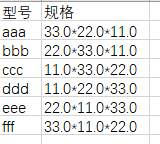解决思路：
将数据读取成Pandas的DataFrame形式，然后采用字符串分割的方法对规格列的内容进行分割，并添加长，宽，高三列。
app = xw.App(visible=True, add_book=False)
file_list = os.listdir(file_path)
for file in file_list:
if '~$' in file: continue if file.split('.')[-1] != 'xlsx': continue wb = app.books.open(file_path+'\\'+file) for sheet in wb.sheets: values = sheet.range('A1').expand().options(pd.DataFrame, header = 1, index = False).value if values['规格'].empty: continue newValue = values['规格'].str.split('*', expand = True) values['长(mm)'] = newValue values['宽(mm)'] = newValue values['高(mm)'] = newValue values.drop(['规格'],axis=1, inplace = True) sheet['A1'].options(index = False).value = values print(values) wb.save() wb.close() app.quit()  任务2：将表格多列内容进行合并 将如下格式的表格中的长，宽，高三列进行合并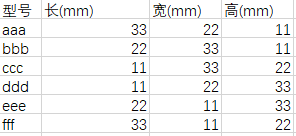解决思路： 将数据读取成Pandas的DataFrame形式，然后采用字符串合并的方法对长，宽，高三列内容进行字符串合并。 app = xw.App(visible=True, add_book=False) file_list = os.listdir(file_path) for file in file_list: if '~$' in file:
continue
if file.split('.')[-1] != 'xlsx':
continue
wb = app.books.open(file_path+'\\'+file)
for sheet in wb.sheets:
values = sheet.range('A1').expand().options(pd.DataFrame, header=1, index=False).value
if values['长(mm)'].empty or values['宽(mm)'].empty or values['高(mm)'].empty:
continue
values['规格'] = values['长(mm)'].astype('str') + '*' + values['宽(mm)'].astype('str') + '*' + values['高(mm)'].astype('str')
values.drop(['长(mm)', '宽(mm)', '高(mm)'], axis=1, inplace=True)
sheet.clear()
sheet['A1'].options(index=False).value = values
sheet.autofit()
wb.save()
wb.close()
app.quit()

备注：

在本任务中，需要用新的数据覆盖原有的数据，在上面使用sheet.clear()来清除原有的数据
Pandas的DataFrame数据是带了序号的，在往excel表格中写入时如果不期望将这个序号写入，则需要使用options(index=False)来指示不带这个序号
表格中读出的数字不是字符串类型，因此在进行合并时需要进行类型转换，使用astype(‘str’)来指示按照str的类型来进行处理


展开全文excel xlwings
• #cell = sheet.cell_value(i,0) # 注意0表示第1数据，1表示第二数据 branch.append(sheet.cell_value(i,0)) #获取表中第一数据 customer_type.append(sheet.cell_value(i,4)) lock_code.append(sheet.cell_...
import numpy as np
import xlrd
import datetime
import time
import numpy as np
import pandas as pd
fp=‘D:/乔/工作簿5.xlsx’#原表的存储路径
worksheet = xlrd.open_workbook(fp)
sheet_names= worksheet.sheet_names()
#存储锁号
lock_code=[]
branch=[]
big_customer=[]
customer_type=[]
for sh in range(3,7):
sheet = worksheet.sheet_by_name(sheet_names[sh])
rows = sheet.nrows # 获取行数
for i in range(1,rows) :
#cell = sheet.cell_value(i,0) # 注意0表示第1列数据，1表示第二列数据
branch.append(sheet.cell_value(i,0))  #获取表中第一列数据
customer_type.append(sheet.cell_value(i,4))
lock_code.append(sheet.cell_value(i,15))
big_customer.append(sheet.cell_value(i,16))

zone=[]
lock=[]
for i in range(len(branch)):
if big_customer[i]==“否” and customer_type[i]!=“中介” and customer_type[i]!=“算量工作室” and customer_type[i]!=“财审” and customer_type[i]!=“监理”:
zone.append(branch[i])
lock.append(lock_code[i])
count={}
temp={}
h=list(temp.fromkeys(zone))
for i in zone:
count[i]=[]
zone_lock={}
for i in range(len(lock)):
count[zone[i]].append(lock[i])
print(count)
temp={}
for i in count.keys():
temp1=list(temp.fromkeys(count[i]))
count[i]=len(temp1)
print(count)
writer = pd.ExcelWriter(“新建.xlsx”)
data = pd.DataFrame(count,index=)
写入Excel文件，路径可以任意指定
data.to_excel(writer, ‘page_1’, float_format=’%.5f’)
writer.save()
writer.close()


展开全文EXCEL sheet
• 我们手里面有很个相同结构的Excel表格，项目经理说这样看起来很不直观，你帮我合并到同一个Excel文件中去，我想了一下有两种实现方式： 原始文件分布截图如下：   一、将个Excel文件通过表单sheet的形式...
      我们手里面有很多个相同结构的Excel表格，项目经理说这样看起来很不直观，你帮我合并到同一个Excel文件中去，我想了一下有两种实现方式：

原始文件分布截图如下：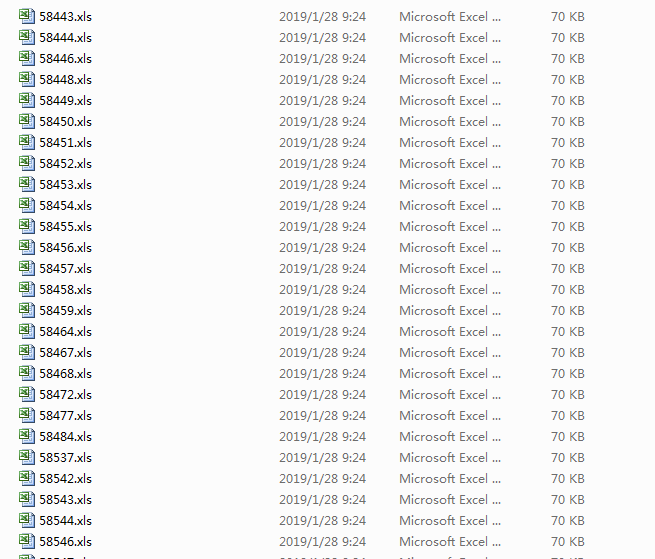一、将多个Excel文件通过多表单sheet的形式合并到同一个Excel文件中去，实现如下：

def trans2Excel(data='Results/',savepath='data.xls'):
'''
将指定目录下所有的Excel文件合并到一个Excel中(多表单sheet的形式)
'''
workbook=xlwt.Workbook(encoding='utf-8')
json_list=os.listdir(data)
for one_json in json_list:
one_json_path=data+one_json
one_code=one_json.split('.').strip()
one_res_data_list=trans2Excel(data_path=one_json_path)
print 'one_res_data_list_length: ',len(one_res_data_list)
i=0
table=workbook.add_sheet(one_code)
for one_list in one_res_data_list:
for j in range(len(one_list)):
table.write(i,j,one_list[j])
i+=1
workbook.save(savepath)

合并后文件截图如下：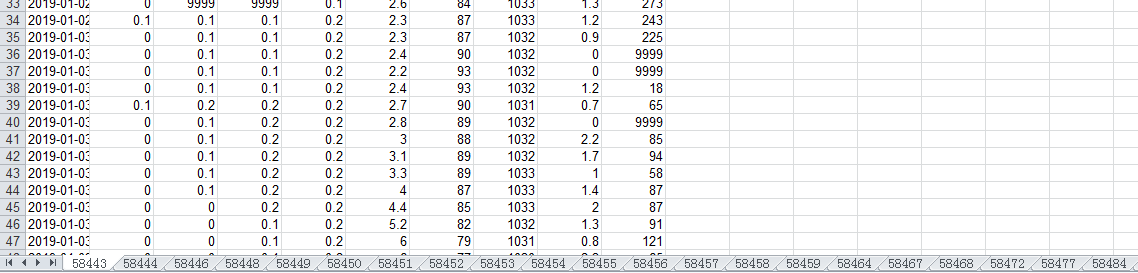从上图可以看到，下方有很多的不同的sheet，就是上述目录中的文件构成的子表单。

二、 通过增加标识列的方式来区分不同Excel文件，进而使用单表单来实现合并，实现如下：

def trans2Excel2(data='Results/',city_path='city.txt',savepath='data.xls'):
'''
不是多表单sheet的形式，而是一个表单，只是增加了标识列
'''
res_list=[['timePoint','rain1h','rain6h','rain12h','rain24h','temperature','humidity','pressure','windSpeed','windDirection','code','name']]
json_list=os.listdir(data)
for one_json in json_list:
one_json_path=data+one_json
one_code=one_json.split('.').strip()
one_res_data_list=trans2Excel2(data_path=one_json_path,city_path=city_path)
print 'one_res_data_list_length: ',len(one_res_data_list)
if one_res_data_list:
res_list+=one_res_data_list
write2Excel(res_list,savepath=savepath)

合并后文件截图如下：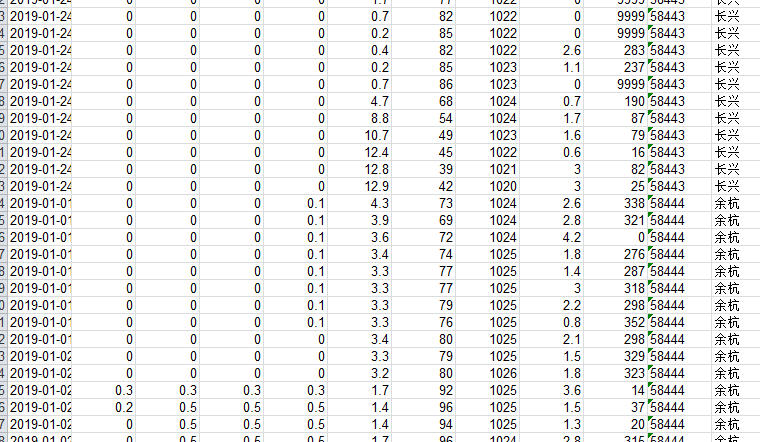最后两列是我们添加的地区和编码的标识列来区分不同地区。

上面两种方式都可以实现多个相同结构Excel文件合并的问题，不同结构的Excel表单文件没有合并的意义。
展开全文• 最近要帮做RA的老姐写个合并excel工作表的脚本……源数据是4000+个excel 工作表，分布在9个xlsm文件里，文件内容是中英文混杂的一些数据，需要从每张表中提取需要的部分，分门别类合并个大的表里。寻觅工具确定...
• numpy操作本地数据及合并多表格一、写入本地数据二、读取本地数据三、合并两个表格 一、写入本地数据 np.savetxt(fname) 参数 意思 fname 文件路径 dtype 数据类型 delimiter 分隔符 fmt 写入文件的...数据分析 numpy
• python合并Excel　---原创，转载请说明　python操作个Excel表格合并为同一个表格的不同sheet，并且将sheet名字命名为原表格的表名　缺陷：Excel表格第一行第一不能空，不然会缺失　速度很慢...
• 在一次数据处理中，需要将900个表格合并成一个表格。每个表格只有两行，第一行是表头（header），第二行是表头的每一项对应的值。这些表格的表头个数和内容都不完全一致，最终的结果表格的表头应当包含所有表格的...
• 实现文件夹内文件遍历并合并所有sheet。 需要解决的问题： １、指定ｓｈｅｅｔ名称 2、除首个文件外， 去掉标题行。 3、在特定添加内容。 #代码为转载 #!/usr/bin/env python # -*- coding: utf-8 -*- # @Author: ...
• 在工作中经常遇到需要将数据输出到excel，且需要对其中一些单元格进行合并，比如如下表表格，需要根据A的值，合并B、C的对应单元格 pandas中的to_excel方法只能对索引进行合并，而xlsxwriter中，虽然提供有...
• 起因： 有一批数据需要每个月进行分析...#读取第一作为合并表格的第一 from pandas import read_csv df = read_csv(r'E:\excel\vb\excel1.csv',header=None) sample_name = df file="combine" filedestinatio
• 如图，手头上有5个xlsx文件，其中“评先得分”中的姓名最多最全，其他表格的姓名包含在“评先得分”中，且这些表格中都只有一个“sheet"，要将“评先得分”与其他4个表格横向合并，找“姓名”公共进行横向拼接。...
• 起因： 有一批数据需要每个月进行分析，数据存储在excel中，行标题一致，需要横向合并进行分析。 数据示意： 具有个 代码： ...# -*- coding: utf-8 -*- ...#读取第一作为合并表格的第一 from pandas imporexcel
• 各位大神好，小弟目前在自学Python处理办公的一些数据，遇到一个问题： 假设目前有一份excel数据，格式为 df = ['ID', 'Name', 'Tracking Number'], 大概几千行这样； 另一个文件夹A下面有名称格式包含生成日期的...
• 任务1：将所有明细表进行合并，并按照产品类别拆分成个页面 解决办法： 第一步：使用Pandas DataFrame的append方法，将所有的明细表中的内容合并到一个表格中 第二步：使用Pandas DataFrame的groupby方法，指定...数据分析 xlwings
• 同时设置一个 excel 个 sheet 页的格式 项目实施过程中，遇到需要设置表格格式的需求，大致有以下几类： 将数值转换为千分位，...将第一日期值一样的合并 设置列宽 设置行高 添加边框 踩过的坑（很重要） 若...RPA
• 前两天有人提了下面这样一个问题，其中一个是“一行拆多行”（将单行一中的个值分成多行单值），另外一个是“多行并一行”（将多行单值合并为单行一中的个值）。这是在对Excel数据表格进行数据处理时经常...编程语言 excel
• Python强大的Pandas库—数据艺术家 ...文章目录Python强大的Pandas库—数据艺术家1、基本操作2、选择数据3、pandas设置值，修改值4、处理丢失数据5、导入导出数据6、合并多个DataFrame7、数据可视化 1、基本操
• 当我们对2个表进行数据合并的时候需要通过指定两个表中相同的作为key，然后通过key匹配到其中要合并在一起的values值。 然后对于merge函数在Pandas中分为1vs1, (m)vs1，以及(m)vs(m)这三种场景。但是平时用...函数
• 目录1.导出excel文件2.隐藏或删除行、3.合并单元格并填入数据4.添加边框5.调整行、列宽6....自从学了python，就方便了，将数据导入用python整理好后，保存为excel文件简直不要太简单，每次有需要的
• 包的安装1.Excel读取1.1读取对应表格1.2读取单元格1.3读取个格子的值1.4练习题2.Excel写入2.1写入单元格并保存2.2写入行数据并保存2.3将公式写入单元格保存2.4插入数据2.5插入行数据2.6删除2.7移动2.8Sheet表...# python表格多列合并python 订阅